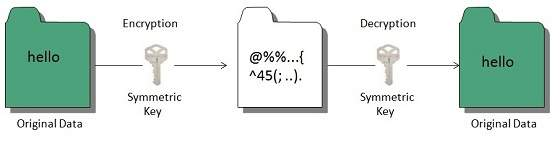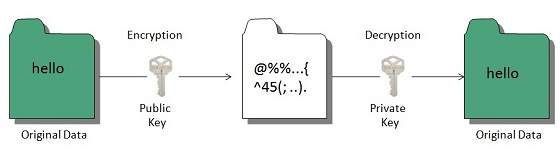# Data Encryption

## Introduction

Encryption is a security method in which information is encoded in such a way that only authorized user can read it. It uses encryption algorithm to generate ciphertext that can only be read if decrypted.

### Types of Encryption

There are two types of encryptions schemes as listed below:

• Symmetric Key encryption

• Public Key encryption

#### Symmetric Key encryption

Symmetric key encryption algorithm uses same cryptographic keys for both encryption and decryption of cipher text.#### Public Key encryption

Public key encryption algorithm uses pair of keys, one of which is a secret key and one of which is public. These two keys are mathematically linked with each other.## Hashing

In terms of security, hashing is a technique used to encrypt data and generate unpredictable hash values. It is the hash function that generates the hash code, which helps to protect the security of transmission from unauthorized users.

### Hash function algorithms

Hashing algorithm provides a way to verify that the message received is the same as the message sent. It can take a plain text message as input and then computes a value based on that message.

Key Points

• The length of computed value is much shorter than the original message.

• It is possible that different plain text messages could generate the same value.

Here we will discuss a sample hashing algorithm in which we will multiply the number of a’s, e’s and h’s in the message and will then add the number of o’s to this value.

For example, the message is “ the combination to the safe is two, seven, thirty-five”. The hash of this message, using our simple hashing algorithm is as follows:

2 x 6 x 3 ) + 4 = 40

The hash of this message is sent to John with cipher text. After he decrypts the message, he computes its hash value using the agreed upon hashing algorithm. If the hash value sent by Bob doesn’t match the hash value of decrypted message, John will know that the message has been altered.

For example, John received a hash value of 17 and decrypted a message Bob has sent as “You are being followed, use backroads, hurry”

He could conclude the message had been altered, this is because the hash value of the message he received is:

(3x4x1)+4 = 16

This is different from then value 17 that Bob sent.